Question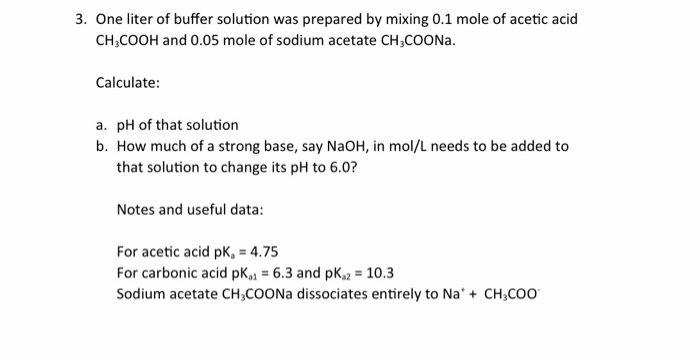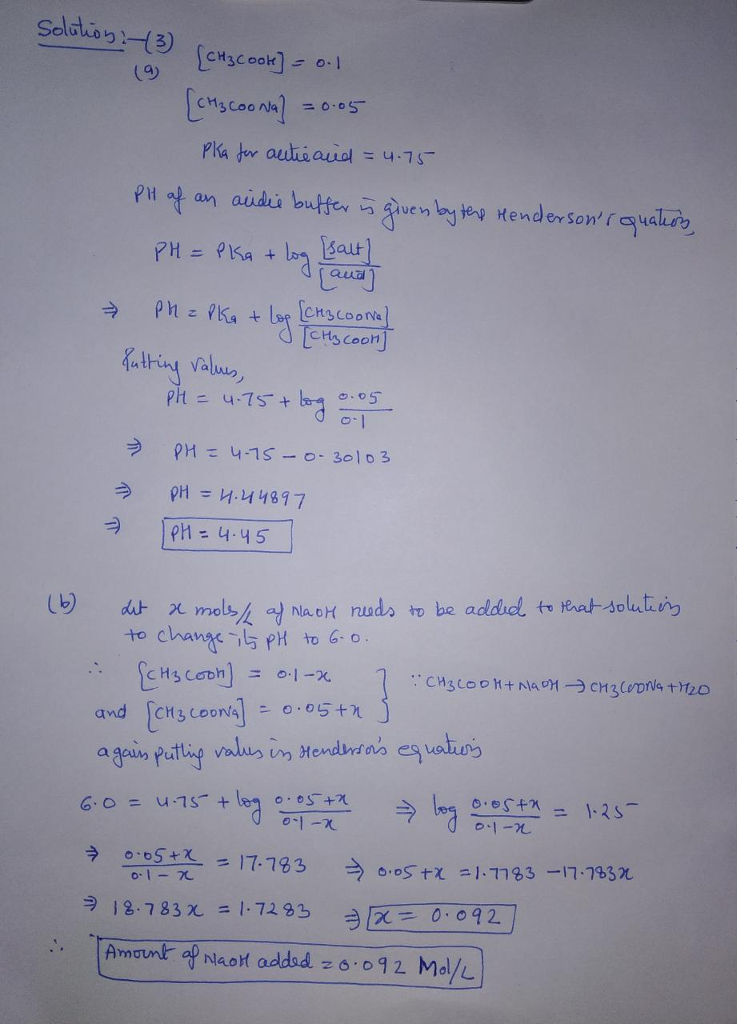#### Earn Coins

Coins can be redeemed for fabulous gifts.

Similar Homework Help Questions
• ### A buffer solution is prepared by mixing 35mL of 0.18M CH3COOH (acetic acid) and 25mL of...

A buffer solution is prepared by mixing 35mL of 0.18M CH3COOH (acetic acid) and 25mL of 0.23M NaCH3COO (sodium acetate). Find the change in pH when 5.0mL of 0.12M of HCL is added. Please show step by step

• ### The pH of a buffer prepared by mixing 0.05 mol of acetic acid (pKa=3.86) with 0.03...

The pH of a buffer prepared by mixing 0.05 mol of acetic acid (pKa=3.86) with 0.03 mol of sodium lactate per liter of aqueous solution is

• ### calculate the ph of a buffer solution that contains 1.5 M acetic acid (CH3COOH) and 0.3...

calculate the ph of a buffer solution that contains 1.5 M acetic acid (CH3COOH) and 0.3 M sodium acetate (CH3COONa) [Ka=1.8x10-5 for acetic acid]

• ### A buffer formed from 20.0 mL of 0.120 M Acetic acid (CH3COOH) (pKa = 4.74) and...

A buffer formed from 20.0 mL of 0.120 M Acetic acid (CH3COOH) (pKa = 4.74) and 12.0 mL of 0.200 M sodium acetate (CH3COONa). What is the pH of this buffer after addition of 0.0001 mol of HCl?

• ### (Please show work) A 100.0 mLbuffer solution is 0.250 M in acetic acid (CH3COOH) and 0.250...

(Please show work) A 100.0 mLbuffer solution is 0.250 M in acetic acid (CH3COOH) and 0.250 M in sodium acetate (CH3COONa). [Acetic Acid (CH3COOH) Ka = 1.8 x 10-5] a)What is the pH of this buffer solution? b)What is the pH after addition of 0.0050 mol of HCl? c)What is the pH after addition of 0.0050 mol of NaOH?

• ### please solve and show work! A buffer contains 0.50 M CH3COOH (acetic acid) and 0.50 M...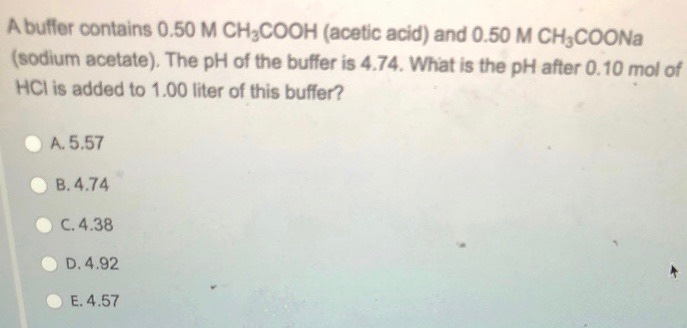please solve and show work! A buffer contains 0.50 M CH3COOH (acetic acid) and 0.50 M CH3COONa (sodium acetate). The pH of the buffer is 4.74. What is the pH after 0.10 mol of HCI is added to 1.00 liter of this buffer? A.5.57 B.4.74 C.4.38 D. 4.92 E.4.57

• ### a buffer solution of pH =5.30 can be prepared by dissolving acetic acid and sodium acetate...

a buffer solution of pH =5.30 can be prepared by dissolving acetic acid and sodium acetate in water. How many moles of sodium acetate must be added to 1 L of 0.25 M acetic acid to prepare the buffer? Ka(CH3COOH)=1.8 x 10^-5

• ### 1. Calculate the pH of a buffer solution prepared by mixing 0.250 L of 0.150 M...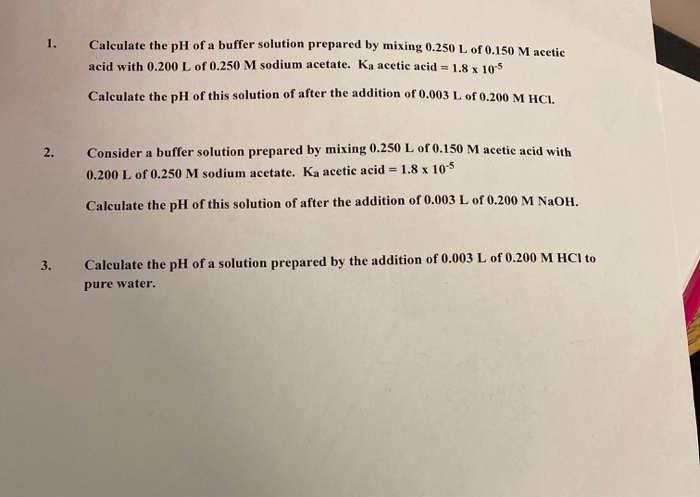1. Calculate the pH of a buffer solution prepared by mixing 0.250 L of 0.150 M acetic acid with 0.200 L of 0.250 M sodium acetate. Ka acetic acid = 1.8 x 10-5 Calculate the pH of this solution of after the addition of 0.003 L of 0.200 M HCL 2. Consider a buffer solution prepared by mixing 0.250 L of 0.150 M acetic acid with 0.200 L of 0.250 M sodium acetate. Ka acetic acid = 1.8 x 10-5...

• ### Calculate the pH of a buffer solution prepared by mixing 75 mL of 1.0 M lactic...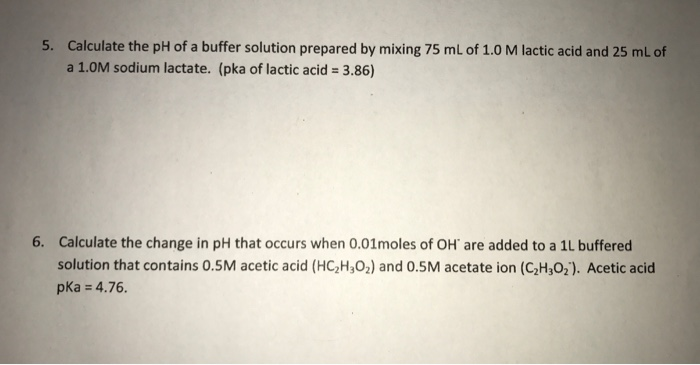Calculate the pH of a buffer solution prepared by mixing 75 mL of 1.0 M lactic acid and 25 mL of a 1.0M sodium lactate. (pka of lactic acid = 3.86) Calculate the change in pH that occurs when 0.01moles of OH are added to a 1L buffered solution that contains 0.5M acetic acid (HC_2H_3O_2) and 0.5M acetate ion (C_2H_3O_2). Acetic acid pka = 4.76.

• ### A buffer solution is prepared by mixing 15.0 mL of 2.20 M Acetic Acid and 30.0...

A buffer solution is prepared by mixing 15.0 mL of 2.20 M Acetic Acid and 30.0 mL of 1.55 M NaC2H3O2. Determine the pH of the buffer. pKa HC2H3O2 = 4.745 pH:_____